# Metric isomorphism

(diff) ← Older revision | Latest revision (diff) | Newer revision → (diff)

of two measure spacesand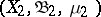; also "strict isomorphism" or "point isomorphism"

2010 Mathematics Subject Classification: Primary: 28Axx [MSN][ZBL]

A bijective mapping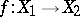for which images and inverse images of measurable sets are measurable and have the same measure (hereis some Boolean-algebra or-ring of subsets of the set, called measurable, and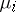is a given measure on). There is the more general notion of a (metric) homomorphism of these spaces (called also measure-preserving transformation), that is, a mappingsuch that inverse images of measurable sets are measurable and have the same measure. For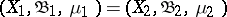, instead of an isomorphism or a homomorphism one speaks of a (metric) automorphism or an endomorphism.

In correspondence with the usual tendency in measure theory to ignore sets of measure zero, there is (and is primarily used) a "modulo 0" version of all these ideas. For example, let,,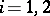, and letbe a metric isomorphism; then it is said thatis an isomorphism modulo 0, or almost isomorphism of the original measure spaces (the stipulation "modulo 0" is often omitted).

For a number of objects given in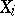(subsets, functions, transformations, and systems of these) one can give a meaning to the assertion that under a metric isomorphismthese objects transform into each other. It is then said thatis a metric isomorphism of the corresponding objects. It is also possible to speak of their being metrically isomorphic modulo 0. This means that for certain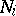of measure zero the corresponding objects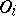may be considered as objects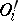in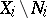(for transformations this means that theare invariant relative to these transformations, whereas for subsets and functions this makes sense for any: take the intersection of the considered subsets withor the restrictions of the functions to) and thatis a metric isomorphism of the objects. A class of all objects metrically isomorphic modulo 0 to each other is called a (metric) type; two objects of this class are said to have the same type.

Associated withare the Hilbert spaces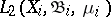in which, in addition to the usual Hilbert space structure, there is also the operation of ordinary multiplication of functions (defined, it is true, not everywhere, since the product of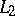functions is not always in), and the Boolean measure σ-algebras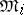, obtained from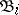by identifying sets with symmetric difference of measure zero (that is, factorizing with respect to the ideal of sets of measure zero). A metric isomorphismmodulo 0 induces an isomorphism of the Boolean measure algebrasand a unitary isomorphism of the Hilbert spaceswhich is also multiplicative, that is, takes a product (whenever defined) to the product of the images of the multiplicands. Ifis a Lebesgue space, then the converse is true: Every isomorphism of the Boolean measure-algebras, or every multiplicative unitary isomorphism of the spaces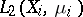, is induced by some metric isomorphism modulo 0.

How to Cite This Entry:
Metric isomorphism. Encyclopedia of Mathematics. URL: http://encyclopediaofmath.org/index.php?title=Metric_isomorphism&oldid=26626
This article was adapted from an original article by D.V. Anosov (originator), which appeared in Encyclopedia of Mathematics - ISBN 1402006098. See original article